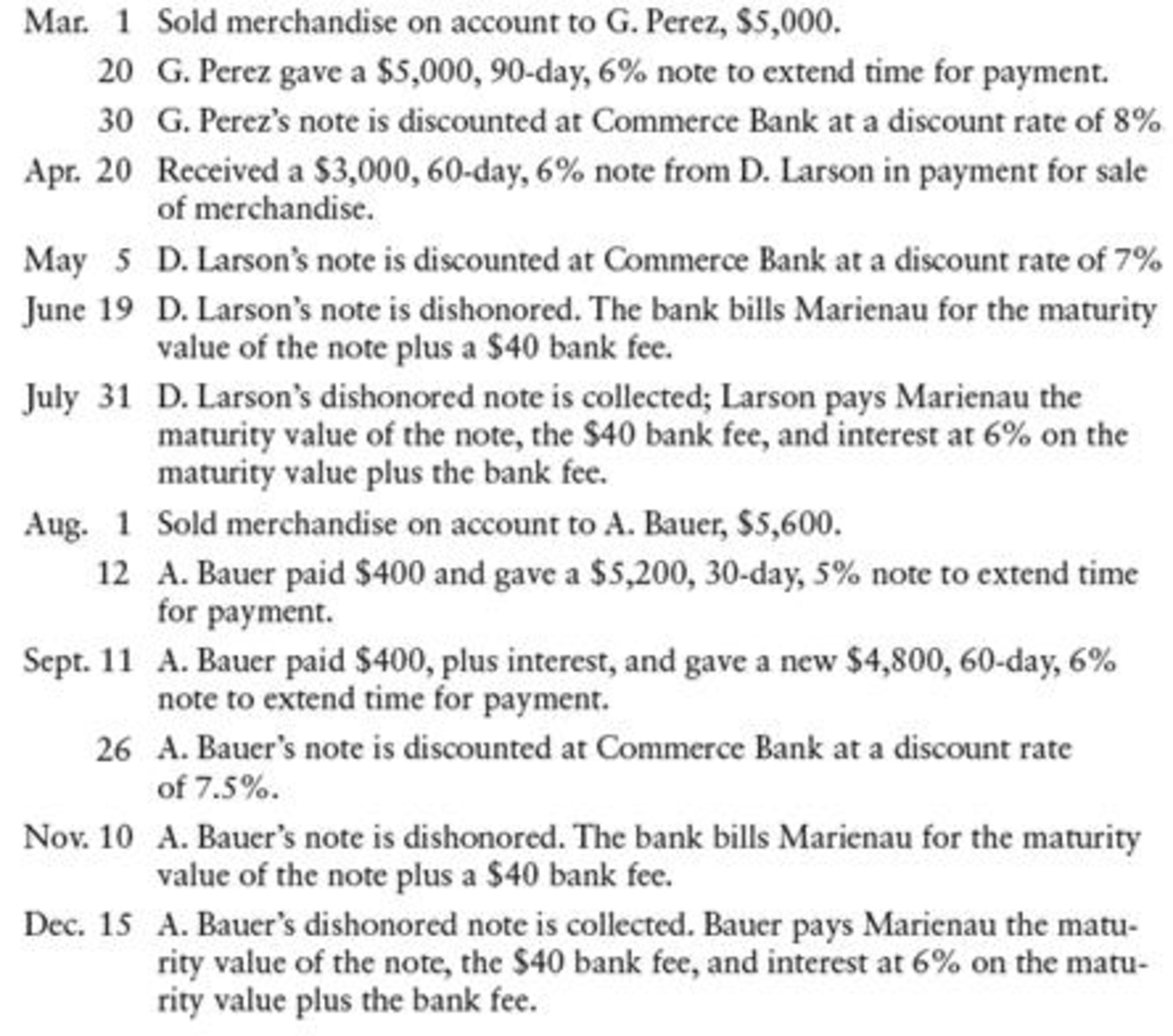Chapter 17, Problem 11SPA

Chapter
Section
Textbook Problem

NOTES RECEIVABLE DISCOUNTING Marienau Suppliers had the following transactions:REQUIREDRecord the transactions in a general journal.

To determine

Prepare a journal entry to record Suppliers M.

Explanation

Note receivable:

Note receivable refers to a written promise for the amounts to be received within a stipulated period of time. This written promise is issued by a debtor or, borrower to the lender or creditor. Notes receivable is an asset of a business.

 Date Account titles and Explanation Debit Credit March 1 Accounts receivable -G.P $5,000 Sales$5,000 (To record sale made on account) March 20 Notes receivable $5,000 Accounts receivable - G.P$5,000 (To record received note to settle  account) March 30 Cash (1) $4,984.78 Interest expense (2)$15.22 Notes receivable $5,000 (To record discount on notes receivable) April 20 Notes receivable$3,000 Sales $3,000 (To record received note for merchandise sale) May 5 Cash (3)$3,003.49 Notes receivable $3,000 Interest revenue (4)$3.49 (To record received payment of note with interest) June 19 Accounts receivable - D. L $3,070 Cash$3,070 (To record cash paid for dishonoured note) July 31 Cash $3,091.49 Accounts receivable - D. L$3,070 Interest revenue (5) $21.49 (To record Collected dishonoured note with interest) August 1 Accounts receivable - A.B$5,600 Sales $5,600 (To record sale made on account) August 12 Cash$400 Notes receivable $5,200 Accounts receivable - A.B$5,600 (To record cash received and note to settle account) September 11 Cash (6) $421.67 Notes receivable (new note)$4,800 Notes receivable (old note) $5,200 Interest revenue (7)$21.67 (To record received new note plus interest on old note) September 26 Cash $4,802.55 Notes receivable$4,800 Interest revenue $2.55 (To record received payment of note with interest) November 10 Accounts receivable - A.B$4,888 Cash $4,888 (To record cash paid for dishonoured note) December 15 Cash$4,916.51 Accounts receivable - A.B $4,888 Interest revenue (8)$28.51 (To record Collected dishonoured note with interest)

Table (1)

Working notes:

(1) Calculate cash proceeds.

Cash proceeds =Maturity value Discount amount (Difference in time period)=($5,000+($5,000×6%×90360))($5,075×8%×80360)=$5,075$90.22=$4,984.78

(2) Calculate interest expense.

Interest expense =Notes payableCash proceeds= $5,000$4,984

Still sussing out bartleby?

Check out a sample textbook solution.

See a sample solution

The Solution to Your Study Problems

Bartleby provides explanations to thousands of textbook problems written by our experts, many with advanced degrees!

Get Started

Find more solutions based on key concepts